Download PDF文章快速检索 高级检索

1. 华东理工大学信息科学与工程学院, 上海 200237;
2. 清华大学自动化系, 北京 100086

Classification algorithm based on Copula theory and Bayesian decision theory
QIAN Dong1, WANG Bei1, ZHANG Tao2, WANG Xingyu1
1. School of Information Science and Engineering, East China University of Science and Technology, Shanghai 200237, China;
2. Department of Automation, Tsinghua University, Beijing 100086, China
Abstract: Traditional Bayesian decision classification algorithm is easily affected by the estimation of class-conditional probability densities, a fact that may result in incorrect classification results. Therefore, this paper proposes an improved classification algorithm based on Bayesian decision, i.e., Bayesian-Copula Discriminant Classifier (BCDC). This method constructs class-conditional probability densities by combining Copula theory and kernel density estimation instead of making assumptions on the form of class-conditional probability densities. Kernel density estimation is used to smooth the probability distribution of each feature. By performing probability integral transform, continuous distribution is converted to random variables having a uniform distribution. Then, Copula functions are used to construct the dependency structure between these probability distributions for two categories. Moreover, the maximum likelihood estimation is applied to determine the parameters of Copula functions, and two well-fitted Copula functions for two categories are selected based on Bayesian information criterion. The BCDC method was validated with experimental datasets of physiological signals. The obtained results showed that the proposed method outperforms other traditional methods in terms of classification accuracy and AUC as well as robustness. Moreover, it takes full advantage of Copula theory and kernel density estimation and improves the accuracy and flexibility of the estimation.
Key words: machine learning     Bayesian decision theory     Copula theory     kernel density estimation     physiological signals

1 Bayesian-Copula 判别分类器 1.1 贝叶斯决策理论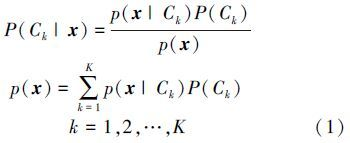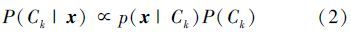1.2 Copula理论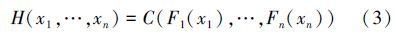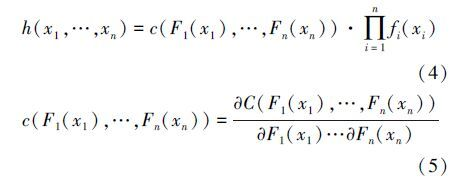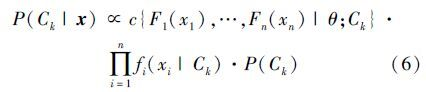Copula函数连接的是每个特征的累积分布函数Fi(xi)，而累积分布函数的值域是[0,1]，因此，当每个特征都是连续的随机变量时，需对数据进行概率积分变换，计算出每个特征的经验累积分布，该方法可以使任意给定的分布转换为均匀分布。

1.3 边缘分布估计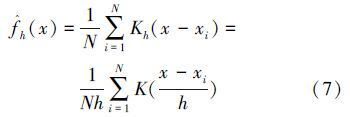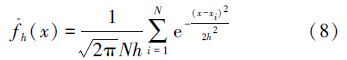1.4 Copula函数参数估计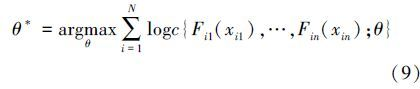1.5 模型选择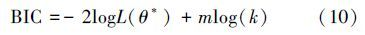2 生物电信号的分类识别

2.1 数据采集

2.2 特征提取

 信号 意义 特征参数 EEG 能量占空比/% Dθ= max{${{{S_\theta }\left( {{C_3}} \right)} \over {{S_T}\left( {{C_3}} \right)}}$×100%,${{{S_\theta }\left( {{C_4}} \right)} \over {{S_T}\left( {{C_4}} \right)}}$×100%} Dα=max{${{{S_\alpha }\left( {{O_1}} \right)} \over {{S_T}\left( {{O_1}} \right)}}$×100%,${{{S_\alpha }\left( {{O_2}} \right)} \over {{S_T}\left( {{O_2}} \right)}}$×100%} EOG 频域能量和/μV2 SLOC(LOC),SROC(ROC)

2.3 模式分类

2.3.1 参数优化和模型选择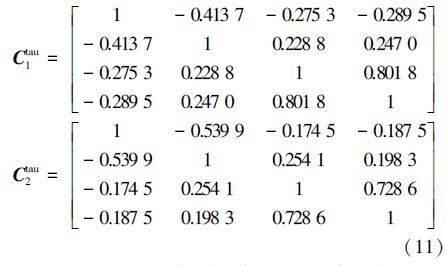Copula密度函数 觉醒状态(A) 困倦状态(D) Gaussian Copula -451.63 -477.25 Student-t Copula -459.46 -471.83

2.3.2 模式分类和模型比较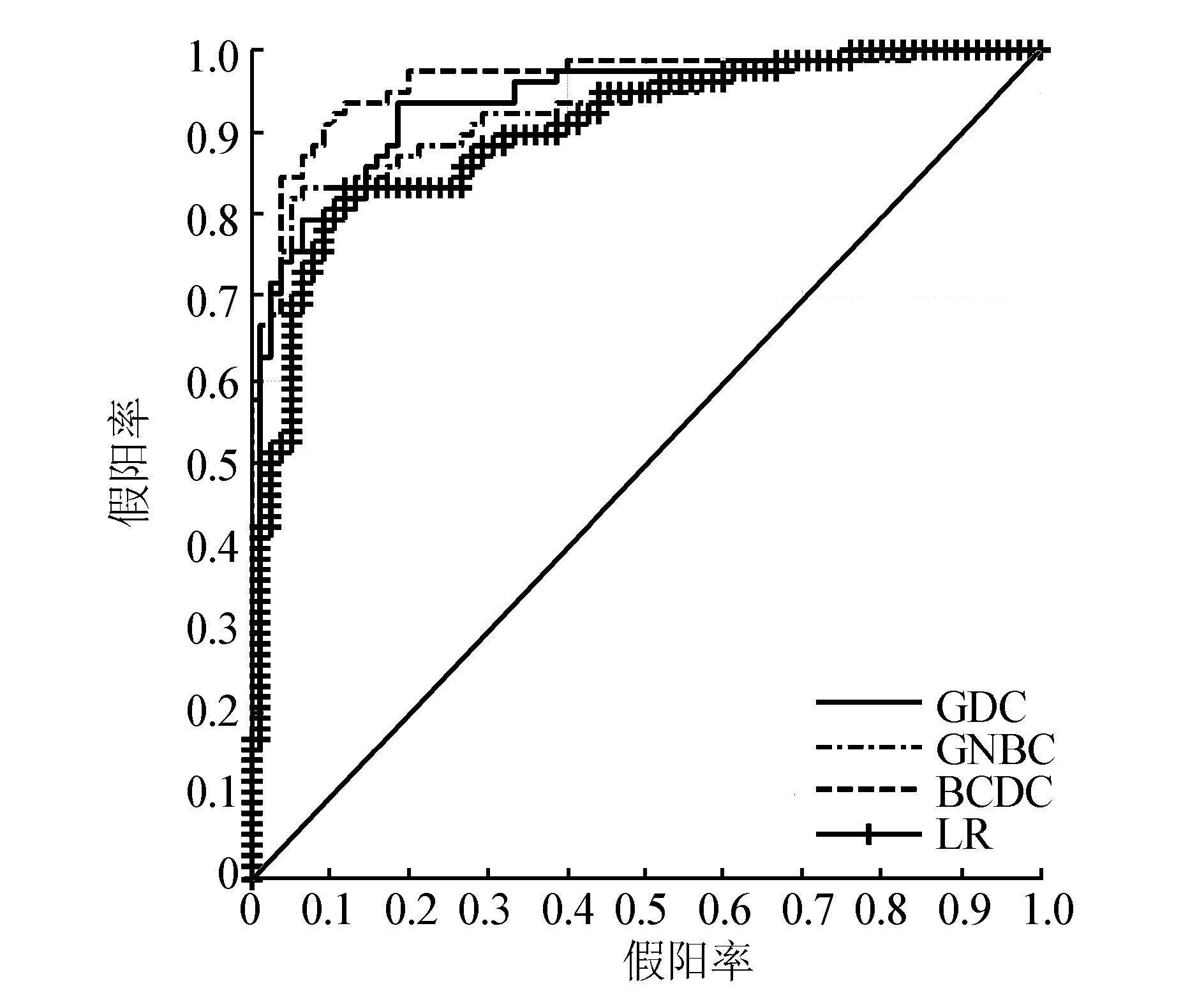图 1 GDC、GNBC、BCDC、LR的ROC曲线 Fig. 1 ROC curves obtained by GDC,GNBC,BCDC,LR,respectively

 分类器 平均精度(标准差) 平均AUC(标准差) GDC 0.855 9(0.025 7) 0.940 8(0.012 9) GNBC 0.858 8(0.025 8) 0.925 3(0.016 9) LR 0.838 2(0.023 9) 0.912 0(0.017 3) BCDC 0.902 6(0.017 9) 0.963 4(0.010 3)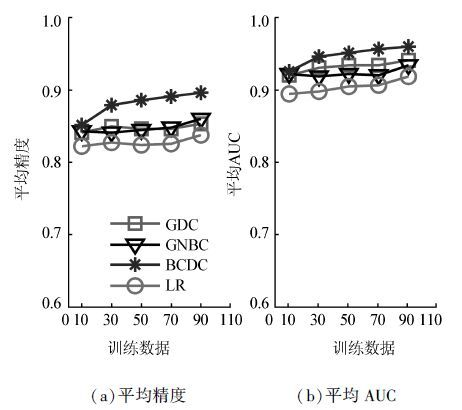图 2 GDC、GNBC、BCDC、LR在不同训练数据个数下的平均精度和平均AUC Fig. 2 Average accuracy and average AUC obtained by GDC,GNBC,BCDC,and LR based on the different subsets of the training data

3 结束语

Copula模型的优势主要是不需要对边缘分布的形式进行假设，在模型中，我们仅仅计算每个特征的经验累积分布，用不同的Copula函数建立特征间的依赖结构。该模型简单、易懂，在对未知数据建立模型时，具有更多的灵活性。对于许多实际问题，概率模型中独立同分布的假设通常是不成立的。所以，通过Copula理论能够提高对联合分布估计的准确性。

  TIPPING M E. Sparse Bayesian learning and the relevance vector machine[J]. Journal of machine learning research, 2001, 1(3):211-244.  XUE Jinghao, HALL P. Why does rebalancing class-unbalanced data improve AUC for linear discriminant analysis?[J]. IEEE transactions on pattern analysis and machine intelligence, 2015, 37(5):1109-1112.  FERNÁNDEZ-DELGADO M, CERNADAS E, BARRO S, et al. Do we need hundreds of classifiers to solve real world classification problems?[J]. Journal of machine learning research, 2014, 15(1):3133-3181.  RODRIGUEZ A, LAIo A. Clustering by fast search and find of density peaks[J]. Science, 2014, 344(6191):1492-1496.  李宏伟, 刘扬, 卢汉清, 等. 结合半监督核的高斯过程分类[J]. 自动化学报, 2009, 35(7):888-895. LI Hongwei, LIU Yang, LU Hanqing, et al. Gaussian processes classification combined with semi-supervised kernels[J]. Acta automatica sinica, 2009, 35(7):888-895.  BLEI D M, NG A Y, JORDAN M I. Latent dirichlet allocation[J]. Journal of machine learning research, 2001, 3(4-5):993-1022.  BISHOP C M. Pattern Recognition and Machine Learning[M]. New York:Springer, 2006:21-31.  NG A Y, JORDAN M I. On discriminative vs. generative classifiers:a comparison of logistic regression and naïve Bayes[C]//Advances in Neural Information Processing Systems. Vancouver, British Columbia, Canada, 2002, 14:841-848.  李航. 统计学习方法[M]. 北京:清华大学出版社, 2012:77-91.  JAIN A K, DUIN R P W, MAO Jianchang. Statistical pattern recognition:a review[J]. IEEE transactions on pattern analysis and machine intelligence, 2000, 22(1):4-37.  DUDA R O, HART P E, STORK D G. Pattern Classification[M]. 2nd ed. New York:Wiley, 2001:20-45.  MURPHY K P. Machine Learning:A Probabilistic Perspective[M]. England:MIT, 2012:82-87.  NELSEN R B. An Introduction to Copulas[M]. 2nd ed. Springer:Berlin, 2006.  GENEST C, FAVRE A C. Everything you always wanted to know about Copula modeling but were afraid to ask[J]. Journal of hydrologic engineering, 2007, 12(4):347-368.  EBAN E, ROTHSCHILD G, MIZRAHI A, et al. Dynamic Copula networks for modeling real-valued time series[C]//Proceedings of the 16th International Conference on Artificial Intelligence and Statistics. Scottsdale, AZ, USA, 2013, 4:247-255.  KRISTAN M, LEONARDIS A, SKOC AJ D. Multivariate online kernel density estimation with Gaussian kernels[J]. Pattern recognition, 2011, 44(10-11):2630-2642.  CHERUBINI U, LUCIANO E, VECCHIATO W. Copula Methods in Finance[M]. England:John Wiley & Sons, 2004.  PATTON A J. A review of Copula models for economic time series[J]. Journal of multivariate analysis, 2012, 110:4-18.  AUBASI A. Classification of EMG signals using PSO optimized SVM for diagnosis of neuromuscular disorders[J]. Computers in biology and medicine, 2013, 43(5):576-586.  TAGLUK M E, SEZGIN N, AKIN M. Estimation of sleep stages by an artificial neural network employing EEG, EMG and EOG[J]. Journal of medical systems, 2010, 34(4):717-725.  CICHOCKI A, MANDIC D, DE LATHAUWER L, et al. Tensor decompositions for signal processing applications:from two-way to multiway component analysis[J]. IEEE signal processing, 2015, 32(2):145-163.  KHUSHABA R N, KODAGODA S, LAL S, et al. Driver drowsiness classification using fuzzy wavelet-packet-based feature-extraction algorithm[J]. IEEE transactions on biomedical engineering, 2011, 58(1):121-131.
DOI: 10.11992/tis.201509011

0

#### 文章信息

QIAN Dong, WANG Bei, ZHANG Tao, WANG Xingyu

Classification algorithm based on Copula theory and Bayesian decision theory

CAAI Transactions on Intelligent Systems, 2016, 11(01): 78-83.
DOI: 10.11992/tis.201509011